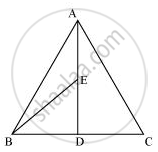# In a triangle ABC, E is the mid-point of median AD. Show that ar (BED) = 1/4ar (ABC). - Mathematics

In a triangle ABC, E is the mid-point of median AD. Show that ar (BED) = 1/4ar (ABC).

#### SolutionAD is the median of ΔABC. Therefore, it will divide ΔABC into two triangles of equal areas.

∴ Area (ΔABD) = Area (ΔACD)

⇒ Area (ΔABD) = 1/2Area (ΔABC)... (1)

In ΔABD, E is the mid-point of AD. Therefore, BE is the median.

∴ Area (ΔBED) = Area (ΔABE)

⇒ Area (ΔBED) = 1/2Area (ΔABD)

⇒ Area (ΔBED) = 1/2*1/2Area (ΔABC) [From equation (1)]

⇒ Area (ΔBED) = 1/4Area (ΔABC)

Concept: Corollary: Triangles on the same base and between the same parallels are equal in area.
Is there an error in this question or solution?
Chapter 9: Areas of Parallelograms and Triangles - Exercise 9.3 [Page 162]

#### APPEARS IN

NCERT Class 9 Maths
Chapter 9 Areas of Parallelograms and Triangles
Exercise 9.3 | Q 2 | Page 162

Share